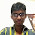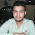## Tuesday, September 21, 2010

### Python and binary data - Part 1

All data is represented by ones and zeroes. How ever, the stream of binary data (ones and zeroes) can represent anything. Practically any thing can be represented with multiple ones and zeroes strung together along with the means for interpretation. The most common interpretation is textual data or Ascii data.
If the representation format is not known then we simply refer to it as binary data.
This interpretation process is called decoding and reverse transformation to binary data is called encoding.
If the binary data is not an ascii representation, you can't manipulate it in a textual editor.
Python has a specific module called 'binascii' for transformation of binary data to ascii representation and back and forth.

So how is a 'A' represented in binary :
>>> ord('A')
65

Note : The value is a decimal representation.


Now we use the binascii to convert the text to ascii binary representation

>>> hexlify('A')
'41'

Note : The value is a hex representation as string.


Lets compare the results:

>>> hex(65)
'0x41'
>>> int('41',16)
65


Now let's do the reverse process:

>>> from binascii import unhexlify
>>> unhexlify('41')
'A'


Since, it returns a string hex value, you may want to convert it to an integer

>>> k = hexlify('Ashish')
>>> k
'417368697368'
>>> m = int(k, 16)
>>> l = hex(m)
>>> l
'0x417368697368'


Converting to binary
>>> from binascii import hexlify
>>> k = hexlify('A')
>>> m = int(k, 16)
>>> bin(m)
'0b101001'


Binary - Hexadecimal representation is easy. Simply make a group of 4 from right to left, padding with zeroes, if necessary.
>>> a = 0x8F7A93
>>> a
9403027
>>> k = bin(a)
>>> k
'0b100011110111101010010011'
>>> k[-4:]
'0011'
>>> k[-8:-4]
'1001'
>>> k[-12:-8]
'1010'


So why would you need binary data?

For many reasons.

The most common being size. Consider if you want to represent "False" and "True". These are 5 bytes long. Simply representing them by one and zero will be just one byte long.
Some thing can not be represented in textual format at all like images etc
Hardware interface so that the binary data can be easily converted to hardware representations like electrical signal.

Number of bits to encode an integer is limited.
Some of the number operations on this limited representation can overflow or exceed the limits on bits used to encode them.

So how are numbers represented by binary data?

1) Unsigned encoding to represent numbers greater than zero
2) Two's complement to represent negative and positive numbers
3) Floating point encoding to represent real numbers

Unsigned encoding is the simplest form of encoding
All numbers can be converted to a binary system of the form = xn * 2**n + x(n-1) * 2 ** (n-1) + x(n-2) * 2** (n-2) + ....
Where xn, x(n-1), x(n-2) .... represents the bit values in 0,1 for position n, n-1, n-2, ... etc
The bit vector xn,x(n-1), x(n-2), .... is unsigned binary encoding representation of a decimal number y. Of course this form of encoding can represent only positive numbers.

In two's complement, the most significant bit is called "signed bit", used to denote negative numbers with 1 and positive numbers with 0. This also means that a 4 bit storage can only use 3 bits to store the number value as significant bit is used to store sign of the number. The other bits are calculated like above. Max value that can be stored is halfed but we could store negative numbers.

A floating point stores numbers of the form + or - p * 2**e. The architecture includes 64 bits - first bit to encode the sign, next 11 bit to encode the exponent e and all other bits (52) to encode the precision p. The exponent is stored as e+ 1023 i.e. a bias of 1023. Since 11 bits can store value from 0 to 2047. This means the exponent can be from -1023 to 1024.
Read here for details on floating point representation.

This explains when does this limited representation breaks and why it is still sufficient for most of us.

1.I googled a lot, and I have to say that this is the best explanation of how Python handles binary data.
Thanks.

2.is really python uses 2's complement to store negative numbers ?

3.Your blog is very useful for me.I really like you post.Thanks for sharing.
goldenslot

4.